# ICSE solutions for Class 10 Mathematics chapter 18 - Trigonometry [Latest edition]

#### Chapters## Solutions for Chapter 18: Trigonometry

Below listed, you can find solutions for Chapter 18 of CISCE ICSE for Class 10 Mathematics.

Exercise 1Exercise 2Exercise 3Exercise 4
Exercise 1

### ICSE solutions for Class 10 Mathematics Chapter 18 Trigonometry Exercise 1

Exercise 1 | Q 1

Solve: 2cos2θ + sin θ - 2 = 0.

Exercise 1 | Q 2

Without using tables evaluate: 3cos 80°. cosec 10° + 2sin 59° sec 31°

Exercise 1 | Q 3

Given that tan (θ_1 + θ_2) = (tan θ_1 + tan θ_2)/(1 - tan θ_1 tan θ_2) Find (θ1 + θ2) when tan θ1 = 1/2 and tan θ_2 = 1/3.

Exercise 1 | Q 4

Without using trigonometric tables, evaluate

sin^2 34^@ + sin^2 56^@ + 2tan 18^@ tan 72^@ - cot^2 30^@

Exercise 1 | Q 5

Without using trigonometric tables, find the value of (sin 72° + cos 18°)(sin 72° - cos 18°).

Exercise 1 | Q 6

Without using tables evaluate: (2tan 53°)/(cot 37°) - (cot 80°)/(tan 10°).

Exercise 1 | Q 7

Solve : Sin2θ - 3sin θ + 2 = 0 .

Exercise 1 | Q 8

If 5 tan θ = 4, find the value of (5 sin θ + 3 cos θ)/(5 sin θ + 2 cos θ)

Exercise 1 | Q 9.1

From trigonometric table, write the values of sin 37°19'.

Exercise 1 | Q 9.2

From the trigonometric table, write the values of cos 23°17'.

Exercise 1 | Q 9.3

From the trigonometric table, write the values of tan 45°48'.

Exercise 1 | Q 10

The string of a kite is 150 m long and it makes an angle of 60° with the horizontal. Find the height of the kite from the ground.

Exercise 1 | Q 11.1

Solve the following equation: (cos θ)/(1 - sin θ) + cos θ/(1 + sinθ) = 4.

Exercise 1 | Q 11.2

Solve the following equation: (cos^2θ - 3 cosθ + 2)/sin^2θ = 1.

Exercise 1 | Q 12.1

Using trigonometric table evaluate the following:
tan 25°45' + cot 45°25'.

Exercise 1 | Q 12.2

Using trigonometric table evaluate the following:
sin 64°42' + cos 42°20'

Exercise 1 | Q 12.3

Using trigonometric table evaluate the following:
cos 64°42' - sin 42°20'

Exercise 1 | Q 12.4

Using trigonometric table evaluate the following:
tan 78°55' - tan 55°18'

Exercise 1 | Q 13.1

(sin 40° + cos 50°)/(tan 38°20')

Exercise 1 | Q 13.2

(sin 20°50' + tan 67°40')/(cos 32°20' - sin 15°10')

Exercise 2

### ICSE solutions for Class 10 Mathematics Chapter 18 Trigonometry Exercise 2

Exercise 2 | Q 1

If x = r sin θ cos Φ, y = r sin θ sin Φ and z = r cos θ, prove that x2 + y2 + z2 = r2

Exercise 2 | Q 2

Prove that (cos θ)/(1 - sin θ) = (1 + sin θ)/(cos θ).

Exercise 2 | Q 3

Prove that tan2Φ + cot2Φ + 2 = sec2Φ.cosec2Φ.

Exercise 2 | Q 4

Prove that sin2 θ + cos4 θ = cos2 θ + sin4 θ.

Exercise 2 | Q 5

Prove that sin4θ - cos4θ = sin2θ - cos2θ
= 2sin2θ - 1
= 1 - 2 cos2θ

Exercise 2 | Q 6

Prove that sqrt((1 - sin θ)/(1 + sin θ)) = sec θ - tan θ.

Exercise 2 | Q 7

(sin A)/(1 + cos A) + (1 + cos A)/(sin A) = 2 cosec A

Exercise 2 | Q 8

Prove that sqrt(2 + tan^2 θ + cot^2 θ) = tan θ + cot θ.

Exercise 2 | Q 9

Prove that (sec θ - 1)/(sec θ + 1) = ((sin θ)/(1 + cos θ ))^2

Exercise 2 | Q 10

Prove that (sin θ tan θ)/(1 - cos θ) = 1 + sec θ.

Exercise 2 | Q 11

Prove that: (sec θ - tan θ)/(sec θ + tan θ ) = 1 - 2 sec θ.tan θ + 2 tan^2θ

Exercise 2 | Q 12

Prove that ( 1 + tan A)2 + (1 - tan A)2 = 2 sec2A

Exercise 2 | Q 13

If x = a sec θ + b tan θ and y = a tan θ + b sec θ prove that x2 - y2 = a2 - b2.

Exercise 2 | Q 14

Prove the following trigonometric identities.

1/(sec A - 1) + 1/(sec A + 1) = 2 cosec A cot A

Exercise 2 | Q 15

Prove that: 2(sin6θ + cos6θ) - 3 ( sin4θ + cos4θ) + 1 = 0.

Exercise 2 | Q 16

Prove that ( 1 + sin θ)/(1 - sin θ) = 1 + 2 tan θ/cos θ + 2 tan^2 θ .

Exercise 2 | Q 17

Prove that : 1 - (cos^2 θ)/(1 + sin θ) = sin θ.

Exercise 2 | Q 18

If A = 30°, verify that sin 2A = (2 tan A)/(1 + tan^2 A).

Exercise 2 | Q 19

Prove that : (sin(90° - θ) tan(90° - θ) sec (90° - θ))/(cosec θ. cos θ. cot θ) = 1

Exercise 2 | Q 20

Prove that cot θ. tan (90° - θ) - sec (90° - θ). cosec θ + 1 = 0.

Exercise 2 | Q 21

Prove that sec θ. cosec (90° - θ) - tan θ. cot( 90° - θ ) = 1.

Exercise 2 | Q 22

Prove that sec2 (90° - θ) + tan2 (90° - θ) = 1 + 2 cot2 θ.

Exercise 2 | Q 23

Prove that cosec2 (90° - θ) + cot2 (90° - θ) = 1 + 2 tan2 θ.

Exercise 2 | Q 24

Prove that (tan θ)/(cot(90° - θ)) + (sec (90° - θ) sin (90° - θ))/(cosθ. cosec θ) = 2.

Exercise 2 | Q 25

Prove that sin( 90° - θ ) sin θ cot θ = cos2θ.

Exercise 2 | Q 26

Prove that sin θ sin( 90° - θ) - cos θ cos( 90° - θ) = 0

Exercise 2 | Q 27

Prove that sin (90° - θ) cos (90° - θ) = tan θ. cos2θ.

Exercise 2 | Q 28

Prove that (sin (90° - θ))/cos θ + (tan (90° - θ))/cot θ + (cosec (90° - θ))/sec θ = 3.

Exercise 2 | Q 29

If A = 60°, B = 30° verify that tan( A - B) = (tan A - tan B)/(1 + tan A. tan B).

Exercise 2 | Q 30

If 2 sin A – 1 = 0, show that: Sin 3A = 3 sin A – 4 sin3A

Exercise 2 | Q 31

If A, B, C are the interior angles of a triangle ABC, prove that \tan \frac{B+C}{2}=\cot \frac{A}{2}

Exercise 2 | Q 32

The length of a shadow of a tower standing on a level plane is found to be 2y meters longer when the seen's altitude is 30° than when it was 45° prove that the height of the tower is y ( √3 + 1 ) meter.

Exercise 2 | Q 33.1

Prove that: sqrt((1 - cos θ)/(1 + cos θ)) = cosec θ - cot θ.

Exercise 2 | Q 33.2

Prove that sqrt((1 + sin θ)/(1 - sin θ)) = sec θ + tan θ.

Exercise 2 | Q 34

If tan A + sin A = m and tan A - sin A = n, then show that m2 - n2 = 4 sqrt(mn).

Exercise 2 | Q 35

If tan α = n tan β, sin α = m sin β, prove that cos2 α  = (m^2 - 1)/(n^2 - 1).

Exercise 2 | Q 36

If cos θ + sin θ = √2 cos θ, show that cos θ - sin θ = √2 sin θ.

Exercise 2 | Q 37

Prove that (cosec A - sin A)( sec A - cos A) sec2 A = tan A.

Exercise 2 | Q 38

Prove that (sin θ tan θ)/(1 - cos θ) = 1 + sec θ.

Exercise 2 | Q 39

Prove that (tan^2 theta)/(sec theta - 1)^2 = (1 + cos theta)/(1 - cos theta)

Exercise 2 | Q 40.1

Prove that (cot A + cosec A - 1)/(cot A - cosec A + 1) = (1 + cos A)/sin A

Exercise 2 | Q 40.2

Prove that ( tan A + sec A - 1)/(tan A - sec A + 1) = (1 + sin A)/cos A.

Exercise 2 | Q 41

Prove that ((1 - cos^2 θ)/cos θ)((1 - sin^2θ)/(sin θ)) = 1/(tan θ + cot θ)

Exercise 2 | Q 42

Prove that sqrt((1 + cos A)/(1 - cos A)) = (tan A + sin A)/(tan A. sin A)

Exercise 2 | Q 43

If secθ + tanθ = p, show that (p^{2}-1)/(p^{2}+1)=\sin \theta

Exercise 2 | Q 44

Prove that (sin A + cos A)/(sin A - cos A) + (sin A - cos A)/(sin A + cos A) = 2/(2 sin^2 A - 1).

Exercise 2 | Q 45

Prove that  sin^2 θ/ cos^2 θ + cos^2 θ/sin^2 θ = 1/(sin^2 θ. cos^2 θ) - 2.

Exercise 2 | Q 46

If x sin3θ + y cos3 θ = sin θ cos θ  and x sin θ = y cos θ , then show that x2 + y2 = 1.

Exercise 2 | Q 47

Prove that tan A/(1 + tan^2 A)^2 + cot A/(1 + cot^2 A)^2 = sin A.cos A

Exercise 2 | Q 48

Prove that sin A/(sec A + tan A - 1) + cos A/(cosec A + cot A - 1) = 1.

Exercise 2 | Q 49

Prove that tan^3 θ/( 1 + tan^2 θ) + cot^3 θ/(1 + cot^2 θ) = sec θ. cosec θ - 2 sin θ cos θ.

Exercise 2 | Q 50

Prove that ((1 + sin θ - cos θ)/( 1 + sin θ + cos θ))^2 = (1 - cos θ)/(1 + cos θ).

Exercise 2 | Q 51

Prove the following trigonometric identities.

cos A/(1 - tan A) + sin A/(1 - cot A)  = sin A + cos A

Exercise 2 | Q 52

Prove the identity (sin θ + cos θ) (tan θ + cot θ ) = sec θ + cosec θ.

Exercise 2 | Q 53.1

Prove that cos θ sin (90° - θ) + sin θ cos (90° - θ) = 1.

Exercise 2 | Q 53.2

Prove that cos θ/sin(90° - θ) + sin θ/cos (90° - θ) = 2.

Exercise 2 | Q 53.3

Prove that (sin θ. cos (90° - θ) cos θ)/sin( 90° - θ) + (cos θ sin (90° - θ) sin θ)/(cos(90° - θ)) = 1.

Exercise 2 | Q 53.4

Proved that cosec2(90° - θ) - tan2 θ = cos2(90° - θ)  +  cos2 θ.

Exercise 2 | Q 54.1

Without using the trigonometric table, prove that
tan 10° tan 15° tan 75° tan 80° = 1

Exercise 2 | Q 54.2

Without using the trigonometric table, prove that
cos 1°cos 2°cos 3° ....cos 180° = 0.

Exercise 2 | Q 55.1

Without using trigonometric table, prove that
cos^2 26° + cos 64° sin 26° + (tan 36°)/(cot 54°) = 2

Exercise 2 | Q 55.2

Without using a trigonometric table, prove that
(cos 70°)/(sin 20°) + (cos 59°)/(sin 31°) - 8sin^2 30° = 0.

Exercise 2 | Q 56

Prove that ((tan 20°)/(cosec 70°))^2 + ((cot 20°)/(sec 70°))^2  = 1

Exercise 2 | Q 57

Prove that (sin 70°)/(cos 20°) + (cosec 20°)/(sec 70°) - 2 cos 70° xx cosec 20° = 0.

Exercise 2 | Q 58

If x = h + a cos θ, y = k + b sin θ.
Prove that ((x - h)/a)^2 + ((y - k)/b)^2 = 1.

Exercise 2 | Q 59.1

If A + B = 90°, show that sec2 A + sec2 B = sec2 A. sec2 B.

Exercise 2 | Q 59.2

If A + B = 90°, show that (sin B + cos A)/sin A = 2tan B + tan A.

Exercise 2 | Q 60.1

Prove the following trigonometric identities

cos theta/(1 - sin theta) = (1 + sin theta)/cos theta

Exercise 2 | Q 60.2

Prove the following identities: sec2 θ + cosec2 θ = sec2 θ cosec2 θ.

Exercise 2 | Q 60.3

Prove the following trigonometric identities.

(1 + sin theta)/cos theta + cos theta/(1 + sin theta) = 2 sec theta

Exercise 2 | Q 60.4

Prove the following identities: cot θ - tan θ = (2 cos^2 θ - 1)/(sin θ cos θ).

Exercise 2 | Q 60.5

Prove the following identities:
1/(sin θ + cos θ) + 1/(sin θ - cos θ) = (2sin θ)/(1 - 2 cos^2 θ).

Exercise 2 | Q 61.1

Prove the following trigonometric identities. (1 - cos A)/(1 + cos A) = (cot A - cosec A)^2

Exercise 2 | Q 61.2

Prove the following identities:

(1 - tan^2 θ)/(cot^2 θ - 1) = tan^2 θ.

Exercise 2 | Q 61.3

Prove that the following identities:
Sec A( 1 + sin A)( sec A - tan A) = 1.

Exercise 2 | Q 61.4

Prove that (tan^2 theta)/(sec theta - 1)^2 = (1 + cos theta)/(1 - cos theta)

Exercise 2 | Q 62.1

Prove the following identity :

(secθ - tanθ)^2 = (1 - sinθ)/(1 + sinθ)

Exercise 2 | Q 62.2

Prove that: sin4 θ + cos4θ = 1 - 2sin2θ cos2 θ.

Exercise 2 | Q 62.3

Prove the following identity :

(1 - sin^2θ)sec^2θ = 1

Exercise 2 | Q 62.4

Prove that: cos^2 A + 1/(1 + cot^2 A) = 1.

Exercise 2 | Q 62.5

Prove that identity:
(sec A - 1)/(sec A + 1) = (1 - cos A)/(1 + cos A)

Exercise 2 | Q 63.1

Prove that:  1/(sec θ - tan θ) = sec θ + tan θ.

Exercise 2 | Q 63.2

Prove that: (sin θ - 2sin^3 θ)/(2 cos^3 θ - cos θ) = tan θ.

Exercise 2 | Q 63.3

Prove that (tan θ + sin θ)/(tan θ - sin θ) = (sec θ + 1)/(sec θ - 1)

Exercise 2 | Q 63.4

Prove that identity:
(sec A - 1)/(sec A + 1) = (1 - cos A)/(1 + cos A)

Exercise 2 | Q 64.1

Prove that: (1 + cot^2 θ/(1 + cosec θ)) = cosec θ.

Exercise 2 | Q 64.2

Prove that: (sin A + cos A)/(sin A - cos A) + (sin A - cos A)/(sin A + cos A) = 2/(sin^2 A - cos^2 A).

Exercise 2 | Q 64.3

Prove the following identity :

((1 + tan^2A)cotA)/(cosec^2A) = tanA

Exercise 2 | Q 64.4

Prove that (sin θ + cosec θ)2 + (cos θ + sec θ)2 = 7 + tanθ + cotθ.

Exercise 2 | Q 65.1

Prove the following identity :

sqrt((1 - cosA)/(1 + cosA)) = sinA/(1 + cosA)

Exercise 2 | Q 65.2

Prove that: 1/(cosec A - cot A) - 1/sin A = 1/sin A - 1/(cosec A + cot A)

Exercise 2 | Q 65.3

Prove that: sin6θ + cos6θ = 1 - 3sin2θ cos2θ.

Exercise 2 | Q 65.4

Prove that:
(cos^3 θ + sin^3 θ)/(cos θ + sin θ) + (cos^3 θ - sin^3 θ)/(cos θ - sin θ) = 2

Exercise 2 | Q 66

Prove that sin2 5° + sin2 10° .......... + sin2 85° + sin2 90° = 9 1/2.

Exercise 2 | Q 67

if x/a cos theta + y/b sin theta = 1 and x/a sin theta - y/b cos theta = 1 prove that x^2/a^2 + y^2/b^2  = 2

Exercise 2 | Q 68

Prove the following identity :

[1/((sec^2θ - cos^2θ)) + 1/((cosec^2θ - sin^2θ))](sin^2θcos^2θ) = (1 - sin^2θcos^2θ)/(2 + sin^2θcos^2θ)

Exercise 2 | Q 69

Prove that : tan A/(1 - cot A) + cot A/(1 - tan A) = sec A.cosec A + 1.

Exercise 3

### ICSE solutions for Class 10 Mathematics Chapter 18 Trigonometry Exercise 3

Exercise 3 | Q 1

In figures, find the length CF.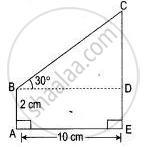Exercise 3 | Q 2

With reference to the given figure, a man stands on the ground at point A, which is on the same horizontal plane as B, the foot of the vertical pole BC. The height of the pole is 10 m. The man's eye s 2 m above the ground. He observes the angle of elevation of C, the top of the pole, as xo , where tan xo =2/5.
Calculate :
(i) the distance AB in metres.
(ii) angle of elevation of the top of the pole when he is standing 15 metres from the pole. Give your answer to the nearest degree.

Exercise 3 | Q 3

From the top of a tower 60 m high, the angles of depression of the top and bottom of pole are observed to be 45° and 60° respectively. Find the height of the pole.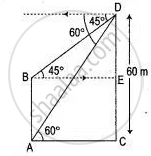Exercise 3 | Q 4

In triangle ABC, AB = 12 cm, LB = 58°, the perpendicular from A to BC meets it at D. The bisector of angle ABC meets AD at E. Calculate:
(i) The length of BD;
(ii) The length of ED.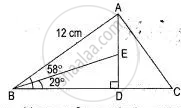Exercise 3 | Q 5

From the top of a lighthouse 100 m high the angles of depression of two ships on opposite sides of it are 48° and 36° respectively. Find the distance between the two ships to the nearest metre.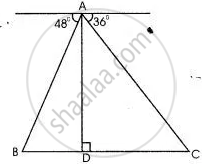Exercise 4

### ICSE solutions for Class 10 Mathematics Chapter 18 Trigonometry Exercise 4

Exercise 4 | Q 1

From a window A , 10 m above the ground the angle of elevation of the top C of a tower is X °, Where tan x ° = 5/2  and the angle of depression of the foot D of the tower is Y° , Where tan y ° = 1/4.  calculate the height CD of the tower in metres .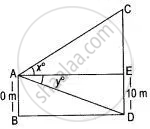Exercise 4 | Q 2

From a lighthouse, the angles of depression of two ships on opposite sides of the lighthouse were observed to be 30° and 45°. If the height of the lighthouse is 90 metres and the line joining the two ships passes through the foot of the lighthouse, find the distance between the two ships, correct to two decimal places.

Exercise 4 | Q 3

A man on the deck of a ship is 10 m above water level. He observes that the angle of elevation of the top of a cliff is 42° and the angle of depression of the base is 20°. Calculate the distance of the cliff from the ship and the height of the cliff.

Exercise 4 | Q 4

A man observes the angle of elevation of the top of a building to be 30°. He walks towards it in a horizontal line through its base. On covering 60 m, the angle of elevation changes to 60°. Find the height of the building correct to the nearest metre.

Exercise 4 | Q 5

A vertical tower stands on a horizontal plane and is surmounted by a flagstaff of height 7 meters. At a point in a plane, the angle of elevation of the bottom and the top of the flagstaff are respectively 30° and 60°. Find the height of the tower.

Exercise 4 | Q 6

A pole being broken by the wind the top struck the ground at an angle of 30° and at a distance of 8m from the foot of the pole. Find the whole height of the pole.

Exercise 4 | Q 7

The shadow of a vertical tower on a level ground increases by 10 m when the altitude of the sun changes from 45° to 30°. Find the height of the tower, correct to two decimal places.

Exercise 4 | Q 8

A man on the top of vertical observation tower observes a car moving at a uniform speed coming directly towards it. If it takes 12 minutes for the angle of depression to change from 30° to 45°, how soon after this will the car reach the observation tower? (Give your answer correct to nearest seconds).

Exercise 4 | Q 9

Two men on either side of a temple 75 m high observed the angle of elevation of the top of the temple to be 30° and 60° respectively. Find the distance between the two men.

Exercise 4 | Q 10

An aeroplane when 3,000 meters high passes vertically above another aeroplane at an instance when their angles of elevation at the same observation point are 60° and 45° respectively. How many meters higher is the one than the other?

Exercise 4 | Q 11

From two points A and B on the same side of a building, the angles of elevation of the top of the building are 30° and 60° respectively. If the height of the building is 10 m, find the distance between A and B correct to two decimal places.

Exercise 4 | Q 12

A man is standing on the deck of a ship, which is 10 m above water level. He observes the angle of elevation of the top of a hill as 60° and the angle of depression of the base of the hill as 30°. Calculate the distance of the hill from the ship and the height of the hill.

Exercise 4 | Q 13

A round balloon of radius 'a' subtends an angle θ at the eye of the observer while the angle of elevation of its centre is Φ. Prove that the height of the centre of the balloon is a sin Φ cosec θ/2.

Exercise 4 | Q 14

Vertical tower is 20m high. A man standing at some distance from the tower knows that the cosine of the angle of elevation of the top of the tower is 0.53. How far is he standing from the foot of the tower?

Exercise 4 | Q 15

Two-person standing on the same side of a tower in a straight line with it measures the angle of elevation of the top of the tower as 25° and 50° respectively. If the height of the tower is 70 m find the distance between the two-person.

Exercise 4 | Q 16

As observed from the top of a 80 m tall lighthouse, the angles of depression of two ships, on the same side of a light house in a horizontal line with its base, are 30° and 40° respectively. Find the distance between the two ships. Give your answer corrected to the nearest metre.

Exercise 4 | Q 17

An aeroplane at an altitude of 250 m observes the angle of depression of two Boats on the opposite banks of a river to be 45° and 60° respectively. Find the width of the river. Write the answer correct to the nearest whole number.

Exercise 4 | Q 18

The angles of elevation of the top of a tower from two points A and B at a distance of a and b respectively from the base and in the same straight line with it are complementary. Prove that the height of the tower is sqrt(ab).

Exercise 4 | Q 19.1

The angle of elevation of a cloud from a point 200 metres above a lake is 30° and the angle of depression of its reflection in the lake is 60°. Find the height of the cloud.

Exercise 4 | Q 19.2

If the angle of elevation of a cloud from a point h meters above a lake is a*and the angle of depression of its reflection in the lake is |i. Prove that the height of the cloud is (h (tan β + tan α))/(tan β - tan α).

Exercise 4 | Q 20

From the top of a hill, the angles of depression of two consecutive kilometer stones, due east, are found to be 30° and 45° respectively. Find the distances of the two stones from the foot of the hill.

Exercise 4 | Q 21

A man standing on the bank of a river observes that the angle of elevation of a tree on the opposite bank is 60°. When he moves 50 m away from the bank, he finds the angle of elevation to be 30°. Calculate:

(i) the width of the river;

(ii) the height of the tree.

## Solutions for Chapter 18: Trigonometry

Exercise 1Exercise 2Exercise 3Exercise 4## ICSE solutions for Class 10 Mathematics chapter 18 - Trigonometry

Shaalaa.com has the CISCE Mathematics Class 10 Mathematics CISCE solutions in a manner that help students grasp basic concepts better and faster. The detailed, step-by-step solutions will help you understand the concepts better and clarify any confusion. ICSE solutions for Mathematics Class 10 Mathematics CISCE 18 (Trigonometry) include all questions with answers and detailed explanations. This will clear students' doubts about questions and improve their application skills while preparing for board exams.

Further, we at Shaalaa.com provide such solutions so students can prepare for written exams. ICSE textbook solutions can be a core help for self-study and provide excellent self-help guidance for students.

Concepts covered in Class 10 Mathematics chapter 18 Trigonometry are Trigonometric Ratios of Complementary Angles, Trigonometric Identities, Heights and Distances - Solving 2-D Problems Involving Angles of Elevation and Depression Using Trigonometric Tables, Trigonometry.

Using ICSE Class 10 Mathematics solutions Trigonometry exercise by students is an easy way to prepare for the exams, as they involve solutions arranged chapter-wise and also page-wise. The questions involved in ICSE Solutions are essential questions that can be asked in the final exam. Maximum CISCE Class 10 Mathematics students prefer ICSE Textbook Solutions to score more in exams.

Get the free view of Chapter 18, Trigonometry Class 10 Mathematics additional questions for Mathematics Class 10 Mathematics CISCE, and you can use Shaalaa.com to keep it handy for your exam preparation.